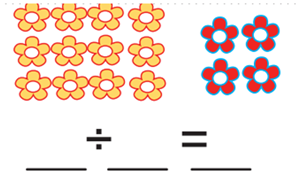Mathematics
Easy

Question

# Find the quotient.## 12 ÷ 4 = 3    12 ÷ 2 = 6    12 ÷ 6 = 2    10 ÷ 5 = 2Hint:

## The correct answer is: 12 ÷ 4 = 3

### 12 ÷ 4 = 33 is the quotient.

Number of yellow flowers = 12
Number of red flowers = 4
If we divide 12 by 4, we get 12/4 = 3 as the quotient
this is a simple division problem.

### Related Questions to study#### With Turito Foundation.#### Get an Expert Advice From Turito.